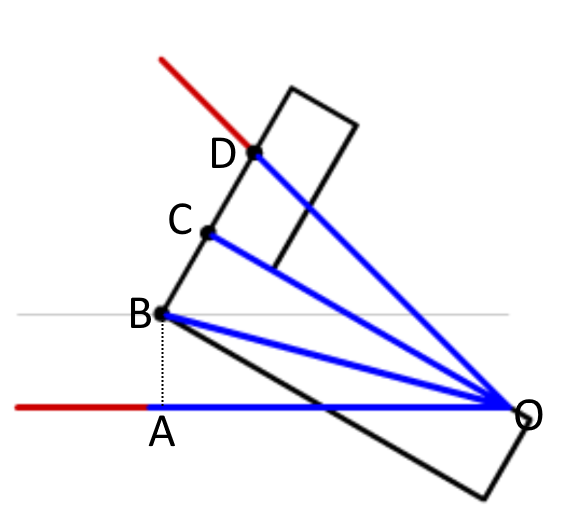#### You may also like### Doodles

Draw a 'doodle' - a closed intersecting curve drawn without taking pencil from paper. What can you prove about the intersections?### Russian Cubes

I want some cubes painted with three blue faces and three red faces. How many different cubes can be painted like that?### Picture Story

Can you see how this picture illustrates the formula for the sum of the first six cube numbers?

# Angle Trisection

##### Age 14 to 16Challenge Level
The diagram below shows the last frame from the video, with some lines and labels added.AB is perpendicular to AO. AB, BC and CD are all equal in length.

Consider triangles OCB and DCO. Since OC is perpendicular to BD (as this is part of the carpenter's square), the two triangles are right-angled. They share a side (OC), and so since BC = CD, they are congruent. Hence angle BOC is equal to angle COD.

Now consider triangle OAB. By construction, AB is perpendicular to AO, so this triangle is also right-angled. It shares a hypotenuse with triangle BOC, and since AB = BC, OAB and OCB are congruent too. So angle AOB is equal to angles BOC and COD, and the angle has been trisected.